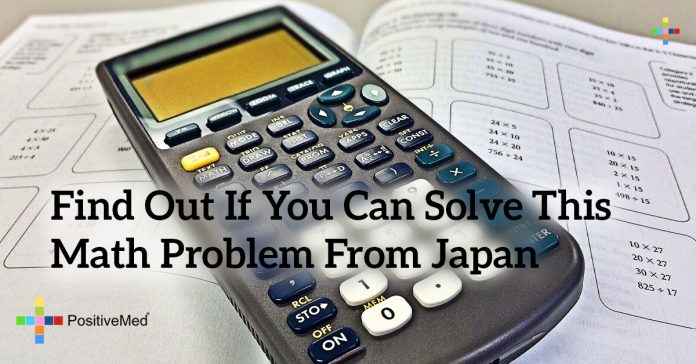# Find Out if You Can Solve this Math Problem From Japan

0
163## Find Out if You Can Solve this Math Problem From Japan

Math is something that many of us find hard. I know. Some of you would use adjectives such as challenging, boring or useless. Still others would prefer descriptions such as educational, interesting or useful. No matter how you describe math, you don’t need to be a genius to solve this math equation.Sadly, many people who try to solve this problem fail to do so correctly. Even sadder, that number is decreasing at an alarming rate. (Of course, that brings us to another branch of math known as statistics!) But, I digress.

What’s important is that people have forgotten how to do basic math. Why? That’s easy. We have been relying on technology for a good many years now and basic math skills are no longer required in many schools across the world.

RELATED ARTICLE: Not Everyone Is Able To See 9 Faces On This Picture, And Here’s Why

If you want to solve this problem, you are going to need to know and understand a math concept known as the Order of Operations. Memorize that and you can solve virtually any math equation out there.

Use this simple acronym to remember what to do first: PEMDAS
1. P (parenthesis)
2. E (exponents)
3. M (multiplication) and D (division)
4. A (addition) and S (subtraction)

If you happen to have more than one of the same level of operation, just start at the left and work towards the right.

Now that you have learned (or refreshed) your math skills, put down that calculator and see if you can solve this problem before watching the video.